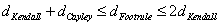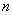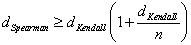## Ordinal Distance

Number usually has order. When we have sequence of number 1, 2, 3, we can say that 3 is higher than 2 and 1, while 2 is higher than 1. When we discuss about nominal scale, we neglect this characteristic of number. When we have categorical data and we assign each set of category to non-arbitrary numbers in an orderly manner, we call this measurement as ordinal scale. Ordinal scale play very important role in behavioral survey because it is relatively easy to design, easy to answer by respondent.

Here are examples of ordinal scale

1. Comparison Index: -2 = strongly disagree, - 1 = disagree, 0 = indifference, 1 = agree, 2 = strongly agree
2. Rating of satisfaction (1 = very dissatisfied, 100 = very satisfied)
3. Rank of priority (1 = best, higher value has lower importance)
4. Ordering (sequence of label based on rank)

A note should be given to distinguish ordering, rank and nominal variable. Both ordering and rank are ordinal variables, though the labels are category. Nominal variable is best represented as existence of the choice, without order . Ordinal variable emphasize the sequence, or order of the choice .

Example :

We have set of fruits: {Grape, Mangoes, Banana, Apple and Orange } and here is my rank of preference and the ordering of my preference

 Rank Ordering Grape = 5 Mangoes = 1 Mangoes = 1 Orange = 2 Banana = 3 Banana = 3 Apple = 4 Apple = 4 Orange = 2 Grape = 5

Given the vector [Grape, Mangoes, Banana, Apple and Orange ], my rank vector is [ 5, 1, 3, 4, 2] or 51342 for short while my ordering vector is [Mangoes, Orange , Banana, Apple, Grape] or MOBAG for short.

To compute dissimilarity or distance between two rank or two ordering or two rating vectors, the most common methods are

Normalized Rank Transformation
Spearman Distance
Footrule Distance
Kendall Distance
Cayley Distance
Hamming Distance
Ulam Distance
Chebyshev /Maximum Distance
Minkowski Distance

Some nice relationship between ordinal distances are given by Marden, 1995 thatIfis the total number of ranks (that we rank 1 as the best andas the worst), thenExcept the first methods (i.e. Normalized Rank Transformation) where we assume rank as quantitative variable, the other methods are utilized special for ordinal variable. Distance for ordinal variables is a measure of spatial disorder between two rank / ordering vectors. We shall name the two rank/ordering vectors as pattern vector and disorder vector . Pattern-vector has order or sequences that disorder-vector want to achieve. Pattern-vector serves as example, guide or goal that the disorder-vector will reach after a number of transformations or operations. Distance for ordinal variables measures the minimum number of operation steps to make disorder-vector into pattern-vector. The different between several distances of ordinal variables are based on the type of operations .

Example:

We asked three persons name Alex, Brian and Cherry their ranking preference over three choices of public transport mode to go to school: Bus, Train and Van. The results are tabulated as follow:

 Judge Ordering Alex {Bus, Van, Train} Brian {Van, Bus, Train} Cherry {Bus, Van, Train}

Distance of preference between Alex and Cherry is zero because they have the same ordering preference. How about distance between Alex and Brian?

Arbitrarily, we can set A = [Bus, Van, Train] as pattern-vector and B=[Van, Bus, Train] as disorder-vector, or we can also set B=[Van, Bus, Train] as pattern-vector and A=[Bus, Van, Train] as disorder-vector. Either ways will give the same result because distance is symmetry: d(A,B) = d(B, A).

Preferable reference for this tutorial is

Teknomo, Kardi (2015) Similarity Measurement. http:\people.revoledu.comkardi tutorialSimilarity